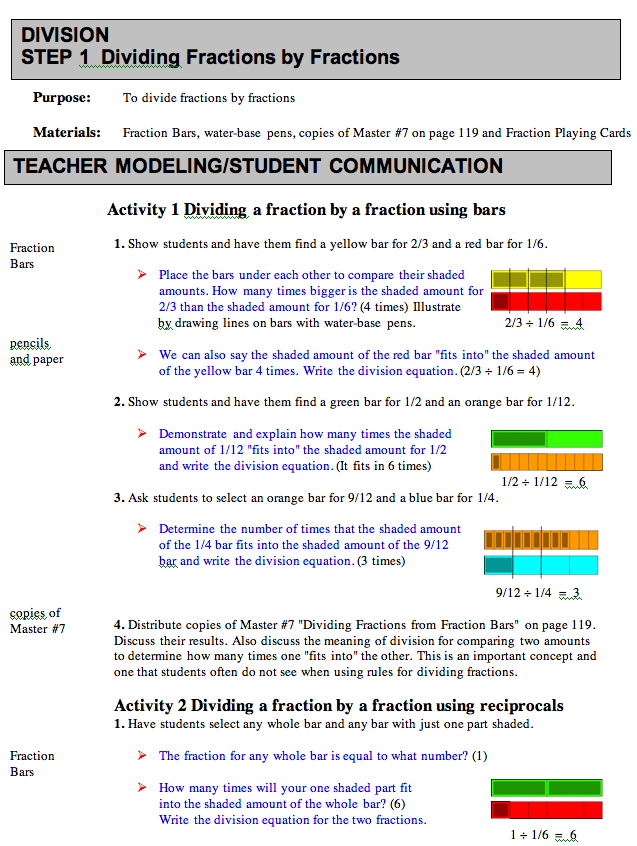Home
 Standards for Grades 3-5, page 152 Examining the effect of multiplying or dividing numbers can also lead to a deeper understanding of these operations. For example, dividing 28 by 14 and comparing the result to 28 by 7 can lead to the conjecture that the smaller the divisor, the larger the quotient. With models or calculators, students can explore dividing by numbers between 0 and 1, such as 1/2, and find that the quotient is larger than the original number. Explorations such as these help dispel common, but incorrect, generalizations such as, "division always makes things smaller."